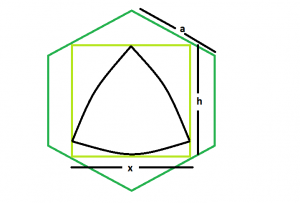GeeksforGeeks App
Open AppBrowser
Continue

# Biggest Reuleaux Triangle inscribed within a square which is inscribed within a hexagon

Given a regular hexagon of side length a which inscribes a square which in turn inscribes a reuleaux triangle. The task is to find the maximum possible area of this reuleaux triangle.
Examples:

```Input: a = 5
Output: 28.3287

Input: a = 9
Output: 91.7848```Approach: As the side of the square inscribed within a hexagon is x = 1.268a. Please refer Largest Square that can be inscribed within a hexagon.
Also, in the reuleaux triangle, h = x = 1.268a
So, Area of the reuleaux triangle, A = 0.70477*h^2 = 0.70477*(1.268a)^2.
Below is the implementation of the above approach:

## C++

 `// C++ Program to find the biggest Reuleaux triangle``// inscribed within in a square which in turn``// is inscribed within a hexagon``#include ``using` `namespace` `std;` `// Function to find the biggest reuleaux triangle``float` `Area(``float` `a)``{` `    ``// side cannot be negative``    ``if` `(a < 0)``        ``return` `-1;` `    ``// height of the reuleaux triangle``    ``float` `h = 1.268 * a;` `    ``// area of the reuleaux triangle``    ``float` `A = 0.70477 * ``pow``(h, 2);` `    ``return` `A;``}` `// Driver code``int` `main()``{``    ``float` `a = 5;``    ``cout << Area(a) << endl;` `    ``return` `0;``}`

## Java

 `// Java Program to find the biggest Reuleaux triangle``// inscribed within in a square which in turn``// is inscribed within a hexagon` `import` `java.io.*;` `class` `GFG``{` `// Function to find the biggest reuleaux triangle``static` `float` `Area(``float` `a)``{` `    ``// side cannot be negative``    ``if` `(a < ``0``)``        ``return` `-``1``;` `    ``// height of the reuleaux triangle``    ``float` `h =(``float``) ``1.268` `* a;` `    ``// area of the reuleaux triangle``    ``float` `A = (``float``)(``0.70477` `* Math.pow(h, ``2``));` `    ``return` `A;``}` `    ``// Driver code``    ``public` `static` `void` `main (String[] args)``    ``{``        ``float` `a = ``5``;``    ``System.out.println( Area(a));``    ``}``}` `// This code is contributed by anuj_67`

## Python3

 `# Python3 Program to find the biggest``# Reuleaux triangle inscribed within``# in a square which in turn is``# inscribed within a hexagon``import` `math` `# Function to find the biggest``# reuleaux triangle``def` `Area(a):` `    ``# side cannot be negative``    ``if` `(a < ``0``):``        ``return` `-``1` `    ``# height of the reuleaux triangle``    ``h ``=` `1.268` `*` `a` `    ``# area of the reuleaux triangle``    ``A ``=` `0.70477` `*` `math.``pow``(h, ``2``)` `    ``return` `A` `# Driver code``a ``=` `5``print``(Area(a),end ``=` `"\n"``)` `# This code is contributed``# by Akanksha Rai`

## C#

 `// C# Program to find the biggest Reuleaux``// triangle inscribed within in a square``// which in turn is inscribed within a hexagon``using` `System;` `class` `GFG``{` `// Function to find the biggest reuleaux triangle``static` `float` `Area(``float` `a)``{` `    ``// side cannot be negative``    ``if` `(a < 0)``        ``return` `-1;` `    ``// height of the reuleaux triangle``    ``float` `h =(``float``) 1.268 * a;` `    ``// area of the reuleaux triangle``    ``float` `A = (``float``)(0.70477 * Math.Pow(h, 2));` `    ``return` `A;``}` `// Driver code``public` `static` `void` `Main ()``{``    ``float` `a = 5;``    ``Console.WriteLine(Area(a));``}``}` `// This code is contributed``// by Akanksha Rai`

## PHP

 ``

## Javascript

 ``

Output:

`28.3287`

Time complexity: O(1)

Auxiliary Space: O(1)

My Personal Notes arrow_drop_up